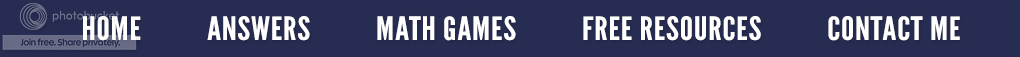### Using Lattice Multiplication in College - A Good or Bad Idea?

I work in the math lab at the college where we teach. All of our students have free access to this tutoring. This is one of my favorite things to do since I am working mostly one-on-one with students. Last week, I had a student who is taking Fundamentals of Algebra. It is a remedial class for those who do not pass the math test to take College Algebra. In this particular class, students are not allowed to use calculators. In other words, it helps if you know your math facts (and sadly, many don’t).

She had to multiply a three-digit number by a two-digit number in one of her word problems. Pretty easy, right? She kept telling me that she could only multiply using the “lettuce” form of multiplication. Many tutors in the lab had no idea what she was talking about; so, I asked her, “Do you mean lattice multiplication?” Once she demonstrated it to the group, I knew that she was using lattice multiplication.

For those of you unfamiliar with it, here is an illustration of what it looks like.
Step #1

Draw a grid so that each digit has its own box. If it is a 3-digit by 3-digit problem, you will need a grid that is three by three. If it is a 3-digit problem by a 2-digit problem, you will need a grid that is three by two.

Step #2

Write the digits for the first factor going across the top of the lattice (grid), one digit per box. Write the second digits on the right going down the lattice, one number per box.

Step #3

Now, divide each box in half by drawing a diagonal line, starting in the top right corner and moving to the bottom left corner. Use a ruler if you like nice straight lines. You can have the diagonal lines continue so that they are outside of the grid boxes. This will help you with your answer!

Step #4

Work through the lattice and multiply each number together. Write the answers in the box. The number in the tens place goes in the upper part of the box, the number in the ones places goes in the lower part of the box. If there is not a number in the tens place, put a zero.

Step #5

To finish, you just add down the diagonal lines. Remember to regroup if necessary

Step #6

Finally, to figure out your product, read the numbers from the left of the grid around to the bottom of the grid.  In the example above, 789 x 461 = 363,729.

This form of multiplication dates back to the 1200s or before in Europe. It gets its name from the fact that to do the multiplication you fill in a grid which resembles a garden lattice, something you might find ivy growing on. Although it works, it is a pretty lengthy process for a college student, especially when she only has so much time to complete a test.

The “vertical” method of multiplication is more efficient because most can write down and solve a 3-digit by 3-digit multiplication problem faster than many students can even draw the lattice. Unfortunately, I showed this student several other ways to multiply, but she failed to grasp their significance. She said that is how she learned in fifth grade, and she was too old to change! With all of that said, lattice multiplication will make it easier for her to transition from lattice multiplication of whole numbers to multiplication of polynomials.